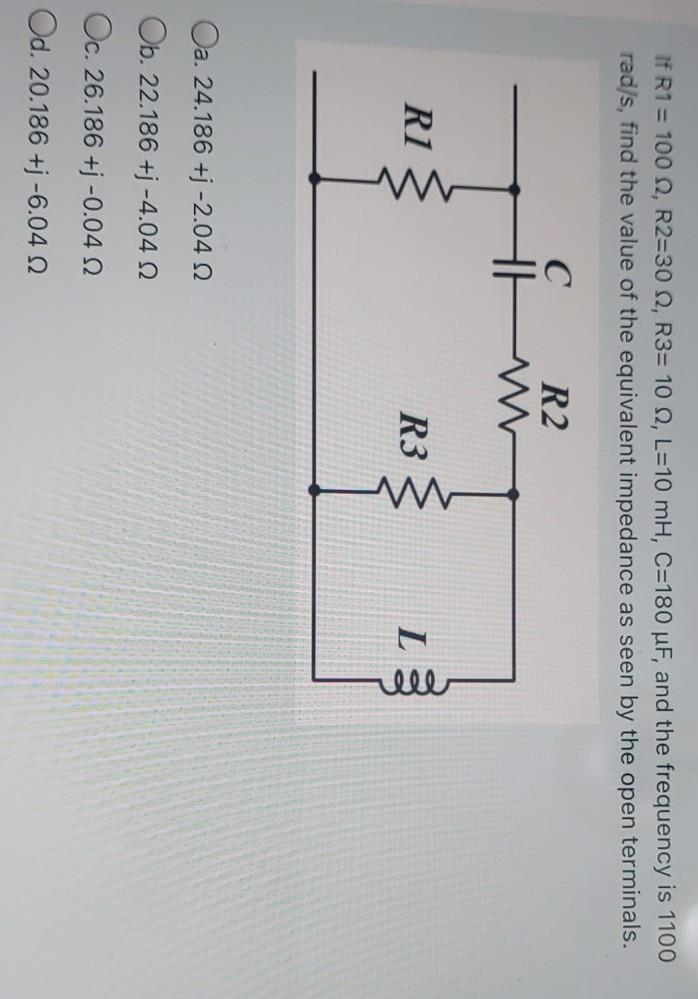### Create an Account

Home / Questions / If R1 = 100, R2=30 12, R3= 10 , L=10 mH, C=180 uF, and the frequency is 1100 rad/s, find t...

# If R1 = 100, R2=30 12, R3= 10 , L=10 mH, C=180 uF, and the frequency is 1100 rad/s, find the value of the equivalent impedance as seen by the open terminals.

If R1 = 100, R2=30 12, R3= 10 , L=10 mH, C=180 uF, and the frequency is 1100 rad/s, find the value of the equivalent impedance as seen by the open terminals. С R2 R1 R3 LS Oa. 24.186 +j -2.041 Ob. 22.186 +j -4.04.12 Oc. 26.186 +j -0.04 12 Od. 20.186 +j -6.042May 31 2021 View more View LessSubscribe To Get Solution